### IMO Shortlist 1961 problem 5

Kvaliteta:
Avg: 0,0
Težina:
Avg: 0,0
Construct a triangle$ABC$ if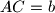$AC=b$,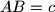$AB=c$ and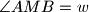$\angle AMB=w$, where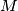$M$ is the midpoint of the segment$BC$ and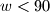$w<90$. Prove that a solution exists if and only if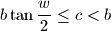In what case does the equality hold?
Izvor: Međunarodna matematička olimpijada, shortlist 1961